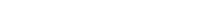# Math riddles Level 23 Answer with Hints and solutionsMath riddles game level 23 Answer with solution android game developed by Black games. Scroll Below to find details.

Math Riddles tests your IQ with mathematical puzzles. Challenge yourself with different levels of math puzzles and stretch the limits of your intelligence. Every IQ game is prepared with an approach of an IQ test. Improve your mathematics, challenge your brain with this game. There are many hard levels which you may not have solved then you can take help from here.

Math app level 23:

49 64 1

9 ? 36

81 25 16Solution: 9 boxes contain squares of 1 to 9, What is missing?

If there is any Doubt/discrepancy then please let me know in comments or you are unable to understand solution then I will surely help you.

### 8 thoughts on “Math Riddles App Level 23 Solution Android”

•July 11, 2019 at 10:24 pm

49 64 1
9 ? 36
81 25 16

the square root of 49 is 7
the square root of 64 is 8
the square root of 1 is 1
the square root of 9 is 3
the square root of 36 is 6
the square root of 81 is 9
the square root of 25 is 5
the square root of 16 is 4

and the missing one is the square root of 2 which is 4.

•May 21, 2019 at 7:46 am

Nah, too ambiguous!
Top row: the difference between 7, the square root of 49, and 8, the square root of 64, is 1. The square of 1 is 1.
Bottom row: the sq.rt. of 81 is 9, the sq.rt. of 25 is 5. Difference is 4, the sq.rt. of 16.
Following this logic the centre square could equally be 81.

•September 6, 2019 at 4:29 pm

word!

•May 15, 2019 at 12:30 pm

only i think the answer is 7？？

•March 3, 2019 at 2:37 pm

Another answer and is totally valid is
The square of 49 minus the square of 64 is -1, squared gives 1

The square of 81 minus the square of 25 is 4, squared gives 16.

Therefore the square of 9 minus the square of 81( the answer) gives -6, squared gives 36.

Thank you.

•January 21, 2019 at 1:00 pm

I dont get it

•January 21, 2019 at 4:05 pm
•